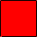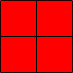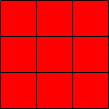Name: sally Who is asking: Other Level: Elementary Question: Is one considered a square number? Hi sally, A square number is a number that is the square of an integer. Thus 4 is a square since 4 is 2 squared and 9 is a square since 9 is 3 squared. Similarly 1 is 1 squared so 1 is a square number. The word square for these numbers comes from geometry. Start with tiles that measure 1 unit by 1 unit.You can make a square from four of these tilesand you can make a square from 9 tiles.. You can also make a square from one tile.Penny Go to Math Central